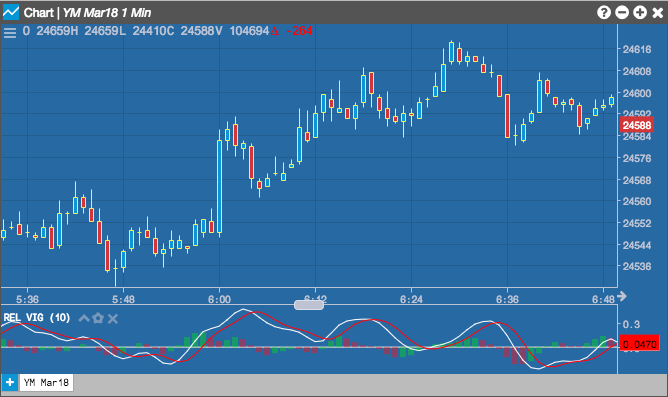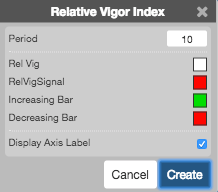Charts

# Relative Vigor Index

The Relative Vigor Index (RVI) is an oscillator that compares an instrument's Close price and Open price to measure the strength of price movements and determine a trend in market activity.## Configuration Options• Period: Number of bars to use in the calculations.
• Color Selectors: Colors to use for graph elements.
• Display Axis Label: Whether to display the most recent value on the Y axis.

## Formula

Usually RVI is displayed as two lines:

$RVI_{1} = \frac{(Close - Open)}{(High - Low)}$

$RVI_{n{\text -}period} = n{\text -}period\; SMA\; of\; RVI_{1}$